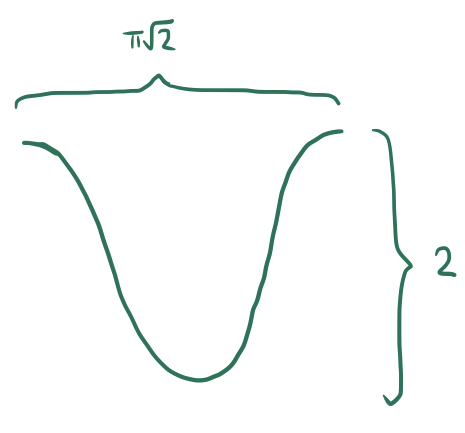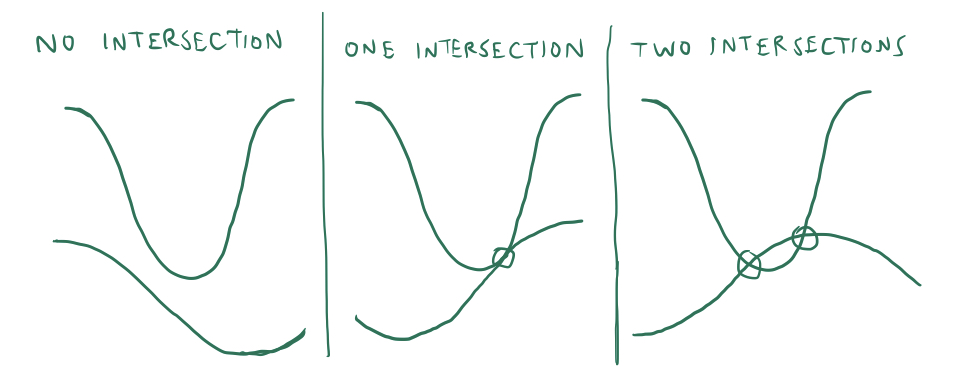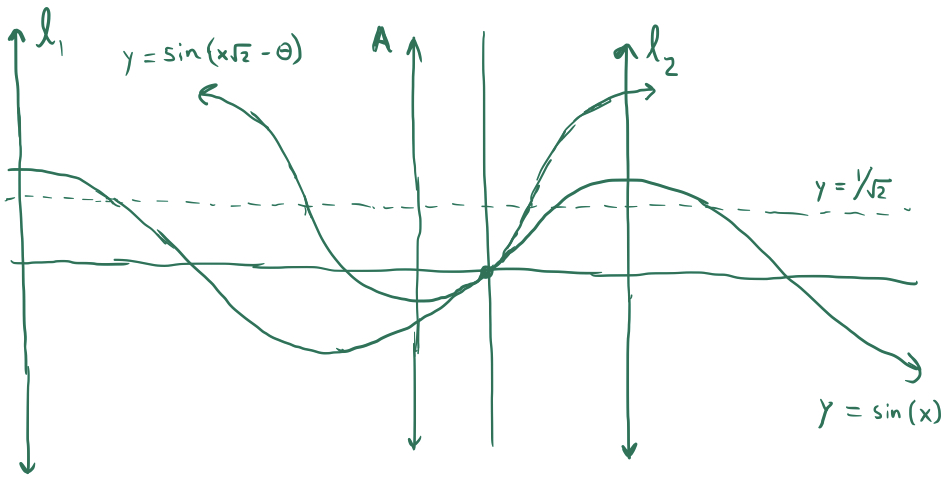## Zeroes of Aperiodic Sinusoids

Consider two sine waves $\sin(x)$ and $\sin(\tau x)$ where $\tau\notin\mathbb Q$. Because $\tau$ is irrational, despite the fact that both sine waves are periodic, their sum $\sin(x)+\sin(\tau x)$ is not periodic. This makes the sinusoid $\sin(x)+\sin(\tau x)$ much more complex than a typical periodic sine wave, and consequentially, much more interesting. In particular, in this post, I will be considering equations of the form where $\tau \notin \mathbb Q$ and $|\alpha| < 2$. However, I won’t be looking for explicit values of solutions $x$ to this equation, since it is unlikely that such solutions exist in closed form for many values of $\tau$ and $\alpha$. Instead, I will consider the distribution of solutions to this equation along the real line, and in particular, the average distance between such solutions (or, the reciprocal of their asymptotic density).

Before I continue, here is a problem for the reader to attempt if he/she/you is/are so inclined:

Find the average distance between consecutive real solutions to the equation and do the same for the equation

We will begin by considering only the average distance between consecutive solutions to and after solving this problem, we will use similar reasoning and techniques to do the same for the general equation for $\tau \notin \mathbb Q$ and $|\alpha|<2$.

Let’s rewrite the equation as If we were to graph the functions of $x$ on the LHS and RHS, we would see an ordinary sine wave with period $2\pi$ centered at $y=0$ and a second, higher-frequency sine wave with period $\pi\sqrt{2}$ centered at $y=\sqrt{1}{\sqrt{2}}$. Solutions to the equation occur when these two curves intersect each other.

Think of the uppermost, higher-frequency curve as an infinite concatenation of inverted “humps”, like this:Each of these humps can interact with the ordinary sine curve below them by intersecting $0$, $1$, or $2$ times:The number of intersections between the humps and the sine curve is determined by how much the inverted hump is “offset” from the maxima (or minima) of the sine curve. In other words: suppose we drew vertical lines $l_1,l_2,...$ through each maximum of $y=\sin(x)$, and another vertical axis $A$ through the minimum of one of the inverted humps of $y=1/\sqrt{2}-\sin(x\sqrt{2})$. If the axis $A$ is very close to one of the axes $l_i$, then the hump will intersect with the sine curve twice, since its minimum will align with the sine curve’s maximum. Similarly, if $A$ is midway between two consecutive $l_i$, then there will be no intersection, since the sine curve will dip downwards as the hump dips downwards, avoiding intersection.

Perhaps we can more precisely formulate when (and how many) intersections will occur. Consider the following diagram:We have an ordinary sine curve $y=\sin(x)$ and a downward “hump” with equation $y=\sin(x\sqrt{2}-\theta)$. The axis $l_2$ is at $x=\pi/2$, and the axis $A$ is at $x=-3\pi/2\sqrt{2}+\theta/\sqrt{2}$, so the horizontal distance between the two axes is $\pi(2+3\sqrt{2})/4-\theta/\sqrt{2}$. I have drawn the two curves in a position so that if $A$ were any closer to $l_2$, there would be two intersections, and if $A$ were a little bit farther away, there would be no intersection. In this configuration, there is only one intersection and the two curves are tangent to each other.

Let $x_0$ be the $x$ value at which these two curves intersect (and are tangent to) each other. First of all, we have that and since they are tangent to each other, their derivatives are also equal, meaning that Note that we now have a system of two equations in $x_0$ and $\theta$. Solving this system yields the neat solutions $x_0=0$ and $\theta=5\pi/4$, meaning that the distance between $A$ and $l_2$ is equal to $\pi(4+\sqrt{2})/8$. This implies that if one of the humps of $y=1/\sqrt{2}-\sin(x\sqrt{2})$ is closer than $\pi(4+\sqrt{2})/8$ to one of the axes of $y=\sin(x)$, it will intersect $y=\sin(x)$ twice, and will otherwise have no intersections unless it is perfectly tangent.

Recall that if $\tau$ is an irrational number, then ${\tau\cdot N}$ behaves like a random variable uniformly distributed in $(0,1)$ if $N$ is chosen uniformly at random from ${-n,-n+1,...,n-1,n}$ and $n\to\infty$. This is very helpful with the problem at hand - it implies that as we consider larger and larger portions of our sinusoid $y=1/\sqrt{2}-\sin(x\sqrt{2})$, the positions of the axes of each inverted hump are distributed uniformly relative to the axes of the sine wave $y=\sin(x)$. If the axis of the hump falls within a distance of $\pi(4+\sqrt{2})/8$ of an axis of the sine wave, then it will result in two intersections, and otherwise it will result in zero intersections (since single intersections occur negligibly often asymptotically). Notice now that is the proportion of the real line that is within $\pi(4+\sqrt{2})/8$ of a maximum of the sine wave $y=\sin(x)$, and so it is also equal to the proportion of inverted humps that intersect with $y=\sin(x)$. Since humps occupy a length of $\pi\sqrt{2}$, the average number of intersections per unit length is equal to The reciprocal of the average number of solutions to our equation per unit length on the real line is equal, of course, to the average distance between consecutive solutions: so we have solved our first problem! Now all we need to do is generalize.

We will follow the same process as before, but this time the period of the elevated sinusoid will equal an arbitrary constant $\tau\notin \mathbb Q$ greater than $1$, and it will be centered around $\alpha$ so that we are now seeking solutions to Once again, we may construct a diagram as we did before with the graphs of the functions $y=\sin(x)$ and $y=\alpha-\sin(\tau x-\theta)$ with the two sinusoids intersecting at a point of tangency at $x=x_0$. Once again, we have the following system of equations: Solving this system is tedious, but it eventually yields the following values: Which means that the maximum distance between the axis of the inverted hump and an axis of the sine wave without two intersections occurring is equal to Plugging in the value of $\theta$ solved for above, we have that the distance is equal to Let $d$ be equal to this distance. Using the same reasoning as before, since $\tau$ is irrational, the distance between the axes behaves randomly and the probability that a random “hump” on the upper sinusoid will intersect the ordinary sine wave twice is equal to and so, since each hump occupies a length of $2\pi/\tau$ on the real line, the average number of intersections per unit length is equal to and so the average distance between zeroes is the reciprocal of this: Plugging in the value of $d$ we obtained earlier, we get the following formula as the final solution to our problem: Keep in mind that this is valid only for $|\alpha|<2$ and $\tau\gt 1$. However, a similar solution for $0\lt \tau\lt 1$ can be obtained easily from this one through scaling.

Now we may easily obtain the average distance between solutions to the equation as requested at the beginning of the post. We can simply set $\tau=\sqrt{2}$ and $\alpha=1$, and plug these values into the above formula to get the following average distance:

I’d like to wrap up this blog post by proposing another similar problem that I haven’t been able to solve yet:

Find the average distance between consecutive real solutions to the equation